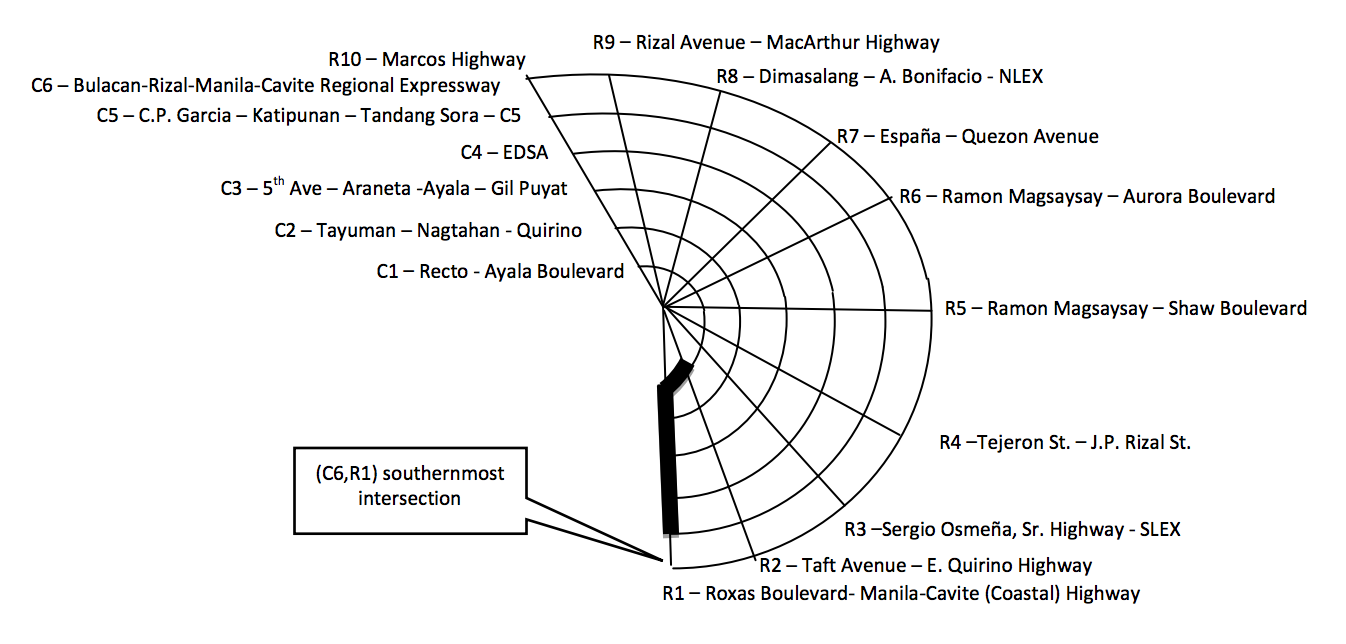시간 제한메모리 제한제출정답맞힌 사람정답 비율
5 초 128 MB0000.000%

## 문제

Metro Manila currently has five circumferential roads arranged in concentric semicircles, with a sixth to be constructed linking the north and the south. From the center (Manila), there are ten radial roads that link the inner and outer areas of Metro Manila.In this problem, we are to find the shortest distance to get from point A to point B. Each point is defined by the intersection of a circumferential and radial road pair (C#, R#) with the center represented as (C0, R0). For calculation purposes, the distance for each segment in the radial road is equal to 1 unit, while each arc is equal to the circumferential road number. For instance, the distance from (C1, R3) to (C2, R3) is one unit, and the distance from (C3, R1) to (C3, R2) is three units. The shortest distance from (C1, R2) to (C5, R1) is five units (See darkened path). It is assumed that C6 is passable for this problem.

However with the new number coding scheme, vehicles are not allowed to use the radial roads with the same last digit of the plate numbers. For instance, a vehicle with plate number of ABC-123 cannot use R3. Furthermore, the circumferential roads (except for C6) also have restrictions. Plate numbers ending in 1 and 2 are not allowed in C1, 3 and 4 in C2, 5 and 6 in C3, 7 and 8 in C4, and 9 and 0 in C5. Thus the shortest path from (C1, R2) to (C5, R1) for vehicle with plate number ABC-123 is five units, but nine units for vehicle with plate number CBA-321. Note that there will be instances that a vehicle cannot reach the destination at all.

## 입력

The input consists of multiple test cases. For simplicity, each test case will have 5 single digit numbers that are whitespace separated. The first two numbers represent the starting point, the next two numbers represent the destination point, and the last number is the ending digit of the plate number. There are no blank lines in between test cases, and the last test case is followed by a line containing a single zero.

## 출력

For each test case, print the case number (starting with 1) followed by the shortest distance needed. Output ‘not possible’ if the destination cannot be reached.

## 예제 입력 1

1 2 5 1 3
1 2 5 1 1
0


## 예제 출력 1

Case 1: 5
Case 2: 9